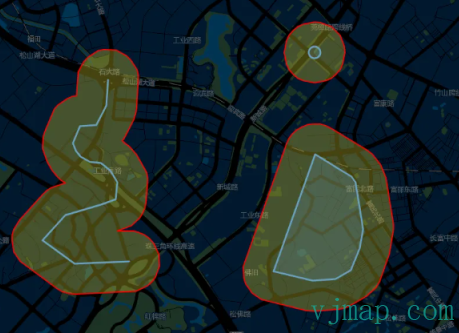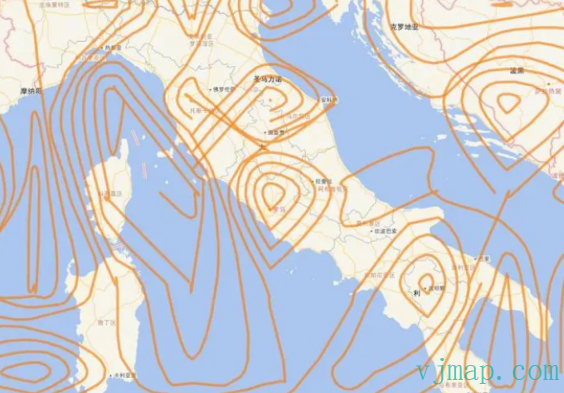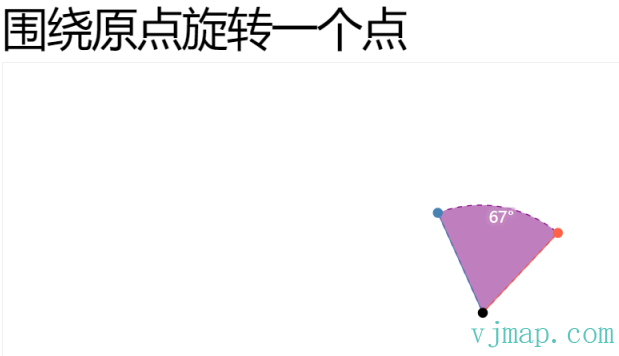# JSTS

JSTS 是一个 ECMAScript 空间谓词和函数库，用于处理符合开放地理空间联盟发布的 SQL 简单特征规范的几何。JSTS 也是成熟的 Java 库JTS的一个端口。

JSTS 是通过 AST 到 AST 转换保留JTS API使用原始 JTS Java 源的自动翻译制作的，除了 I/O 相关类，这些类已被选择性地手动移植并支持 WKT、GeoJSON 和 OpenLayers 3+。

• 几何模型（点、线、面积）

• 几何操作（例如，相交、联合、内部等）

• 几何构造

• 度量函数（例如笛卡尔二维距离、豪斯多夫距离）

• 空间算法（例如缓冲区创建、线偏移、线简化）

• 几何数学函数（如角度、距离等）

• 空间结构（如四叉树和R-树、Delauney三角测量）

• 输入/输出（例如WKT、GML）

• 高精度算术

var reader = new jsts.io.WKTReader()var a = reader.read('POINT (-20 0)')var b = reader.read('POINT (20 0)')// Buffer A and B by 40 unitsa = a.buffer(40)b = b.buffer(40)// Intersection of A and Bvar intersection = a.intersection(b)// Difference of A and Bvar difference = a.difference(b)// Union of A and Bvar union = a.union(b)// Symmetric difference of A and Bvar symDifference = a.symDifference(b)• 功能强大的开源库

• 支持平面坐标系，不限制于地理坐标系

• 文档缺少，示例缺少

# Turf

Turf.js是JavaScript 空间分析库，Turf 实现了常用的空间分析操作，例如生成缓冲区、计算等高线，建立 TIN 等等。以往只属于桌面 GIS 的分析功能，已经可以在浏览器中使用。 Turf 使用 JavaScript 编写，通过 npm 进行包管理。良好的模块化设计使得 Turf 不仅可用于浏览器端，还可以通过 Node.js 在服务器端使用。Turf 原生支持 GeoJSON 矢量数据。GeoJSON 的优点是结构简单，并且得到了所有网页地图API的支持；

github地址： https://github.com/Turfjs/turf

• Measurement 测量计算

• Coordinate Mutation 坐标变换

• Transformation 数据处理

• Feature Conversion 要素转换

• Misc

• Helper 数据工具

• Random 随机生成数据

• Interpolation 插值

• Joins 空间关联

• Grids 网格计算

• Classification

• Aggregation 聚合

• Meta 元数据使用方法

• Assertions 类型校验

• Booleans 布尔类方法

• Unit Conversion 单位换算

var collect = require('@turf/collect');// or in ES6import collect from '@turf/collect';// 使用collect(points, polys, 'population', 'populationValues');​// 全量引用 import * as turf from '@turf/turf'

<script src='https://unpkg.com/@turf/turf@6/turf.min.js'></script><script>    var bbox = turf.bbox(features);</script>

turf缓冲区分析turf 绘制等值线• 功能强大的GIS开源库，持续更新

• 文档全，示例多

• turf.js只支持3857和4326的坐标系。其他坐标系都不支持

# geometric

geometric是一个用于处理几何的 JavaScript 库。非常的小巧。

### 网页浏览器

<script src\="https://unpkg.com/geometric@2.5.0/build/geometric.js"\></script\> <script src\="https://unpkg.com/geometric@2.5.0/build/geometric.min.js"\></script\>

npm i geometric -S

​const geometric = require("geometric");var first_draw = 1;var width = 960, height = 500;var initial_separation = 200;var rect_width = 40, rect_height = 20;​var data = [[-initial_separation + width / 2, height / 2], [initial_separation + width / 2, height / 2]], full_datum, half_datum, full_translate, half_translate;var angle = geometric.lineAngle([data, data]);var full_distance = geometric.lineLength([data, data]);var half_distance = full_distance / 2;

https://bl.ocks.org/HarryStevens/5fe49df19892c04dfb9883c217571409• 小而精

• 代码可读性和理解门槛很低，非常适合用于借鉴其中的代码

• 只有几何计算相关的功能，对GIS空间分析的功能支持不够

# VJMAP

• 点相关算法

• 线相关算法

• 多边形相关算法

• 矩形外包盒相关算法

• 圆和椭圆及曲线相关算法

• 网络拓扑图相关算法

• 等值线分析相关算法• 对一些业务和专业功能进行了一定程序的封装

• 文档较全，示例丰富

• 非开源

• GIS空间分析功能较弱

posted @ 2022-11-15 21:59  vjmap  阅读(149)  评论(0编辑  收藏  举报